# MineSweeper Rules

### A brief guide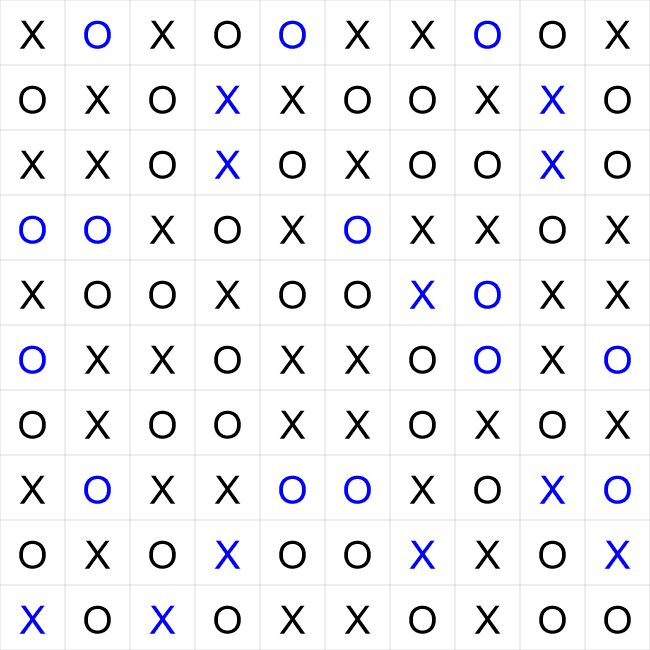This is an example of a completed TicTacToe puzzle. These are the rules,
1. There can't be three or more 'X's or 'O's in a row horizontally or vertically.
2. Each row and column must contain the same number of 'X's and 'O's.
3. It is not allowed to have two identical rows.
4. It is not allowed to have two identical columns.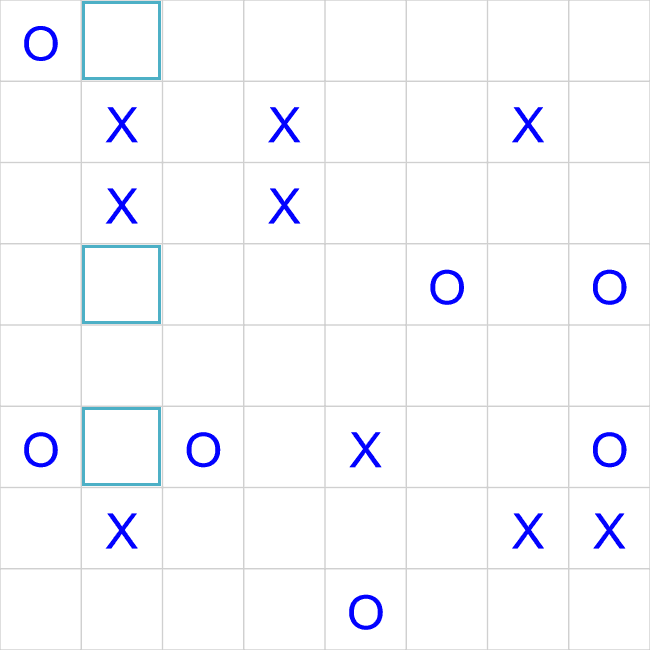This is an example of a TicTacToe starting grid. The start of a TicTacToe puzzle is normally quite easy, there are two things to look for,
• Look for either two 'X's or 'O's in a row (vertically or horizontally). Since it's not allowed to have three (or more) 'X's or 'O's in a row, the cells either side must be the opposite sign. In this grid we have two 'X's in a row, the highlighted cells above and below must be a 'O'.
• Look for two 'X's or two 'O's separated by one empty cell. This empty cell in the middle must be the opposite symbol. If the empty cell wasn't the opposite symbol, we would have three symbols in a row. In this grid we have two 'O's separated by just one cell, this highlighted empty cell must be a 'X'.
We can apply these rules repeatedly in the initial phase of a TicTacToe puzzle.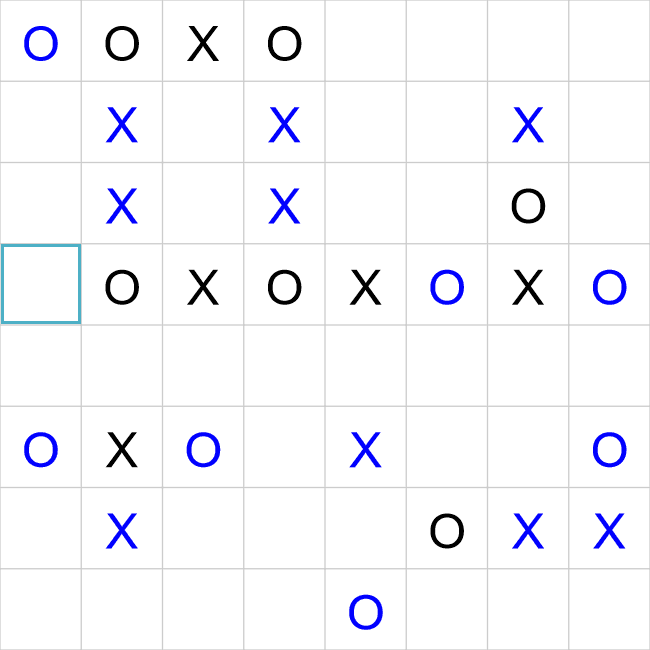We have progressed a little further in this puzzle now, and we have almost filled in a complete row - there is just the single highlighted cell left. By counting the number of 'X's and 'O's already in the rwo we can see we have 3 'X's and 4 'O's. Each row and column must contain the same number of symbols, so the missing highlighted cell must be a 'X'.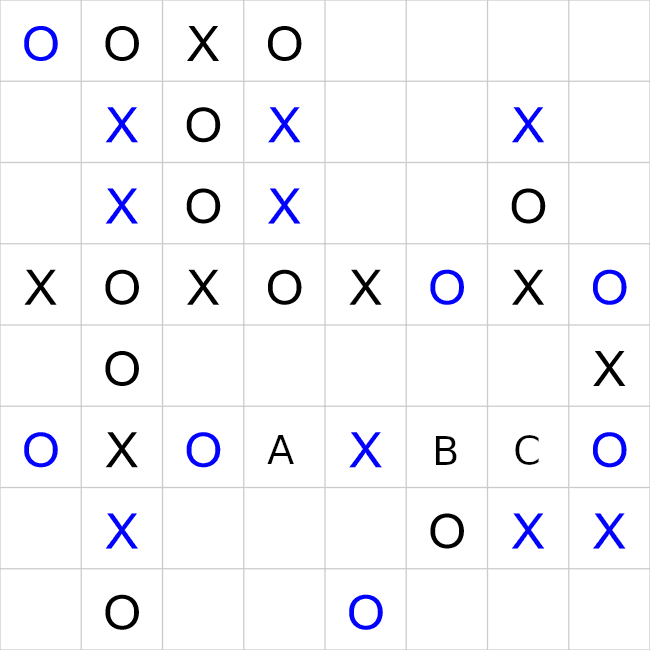You will eventually reach a point in the puzzle where there are no more obvious cells you can fill in. We have reached that point in this puzzle. You should now start looking at the rows/columns that are almost complete.
In this puzzle, look at the 6th row. In this row we have 2 'X's, and 3 'O's, which means the empty cells (marked with 'A', 'B' and 'C') must contain 2 'X' and 1 'O's. Consider where the 'O' could go, if the 'O' went in cell 'A', that would mean that both 'B' and 'C' would be a 'X'. This would give us 3 'X's in a row, which is against the rules of the puzzle, so cell 'A' must be a 'X'.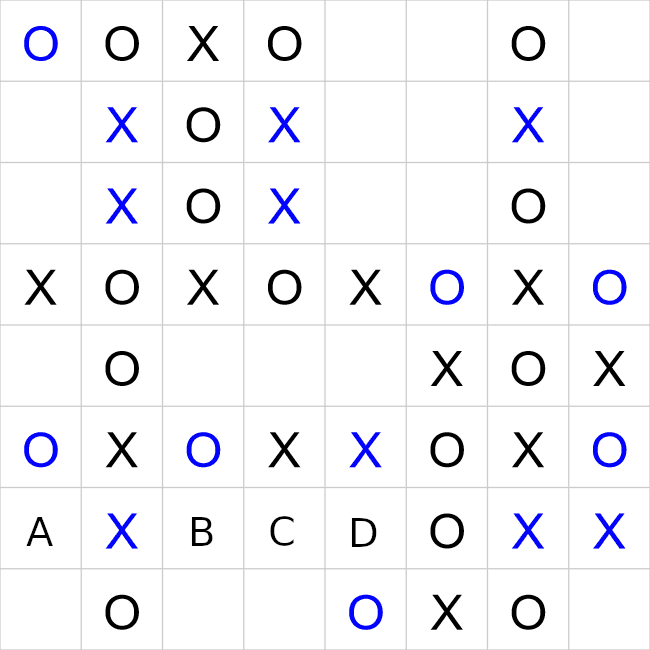We have made a little more progress in this puzzle, but we have now reached another wall. Look at the 7th row, this row already has 3 'X's and only 1 'O's. Consider where the remaining 'X' can't go. It can't go in cell 'A', as that would mean that cells 'B', 'C' and 'D' would be 'O', giving a total of 4 'O's in a row. This is against the rules of the puzzle, so cell 'A' must be a 'O'.
You now have all techniques you need to solve a TicTacToe puzzle. Happy puzzling!